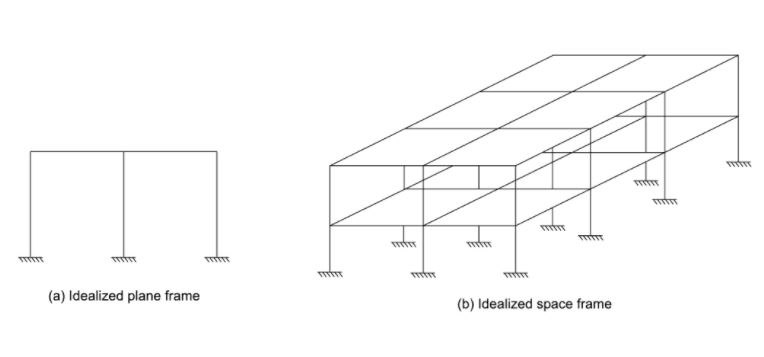# Idealizing a Structure for Structural Analysis

In order perform the structural analysis with ease, we need to idealize a structure into a form without losing the significant accuracy. Generally, they are idealized as 3-dimensional structures. But, in some cases they are idealized as 2D structures that helps to simplify the analysis.

For example, if we consider a beam structure which is 3D element, it is idealized as 2D element, as there is more considered dimension along the horizontal element  (along its span) compared to the other two-dimension ( i.e. along the cross-sectional dimension) as shown in Figure-1.Figure-1: Idealizing an Actual Beam

Similarly, a plane and space frame can be idealized as shown in the figure-2 below.Figure-2: Idealized Plane and Space Frame

### Laws of Superposition

When a structure is subjected to different loads, as per law of superposition, the structure can be divided into several units and the it can be analyzed by summing up the individual structural loads to get final load value. This law of superposition of loads can only be applied when the material is assumed to be perfectly elastic and it follows Hooke's law for the range of loads that is considered.
The law of superposition helps to analyze the structure with convenient end conditions and suitable loadings separately and then combining it together to get the solution for the required end conditions and loadings.

Read More: Law of Superposition in Structural Analysis

### Conditions of Equilibrium

The basic and important tool that is used in structural analysis is the use of equilibrium equations to determine the unknown reactions for a structural element that remains in a stationary position. As per the condition, the reactions from the support and the loads on the structure will remain in static equilibrium.
If so, the conditions of static equilibrium are:
1. The summation of all forces along X, Y and Z-axis is zero.
2. The summation of all the moments about any axis is also zero.
The equations that are derived for static equilibrium are based on the Newton's law.
1. For a 3D system, the equations of equilibrium are: ΣFx =0; ΣFy=0; ΣFz =0; and ΣMx=ΣMy=ΣMz = 0;
2. For a 2D system, the equations of equilibrium are ΣFx=ΣFy=0; ΣMx=ΣMy=0;
The conditions of equilibrium can be explained as per the following example in figure-3. As shown, we have a beam AB, which is subjected to a point load and uniform load along CB. The supports are provided at A and B.

We will consider a single part of the beam AB, i.e. CD. When the portion CD is considered, the loads acting on it are uniform loads, the force Fc and Fd, and the moments Mc and Md.

### Compatibility Conditions in Structural Analysis

A structure is a combination of two of structural elements connected by some type of joints. In a joint, two or more members can meet. The compatibility conditions means the requirement of continuity along the joints.
The compatibility conditions that are satisfied at the joint are:
1. The members that meet at any joint will continue to join at the same junction even after the occurrence of any deformation.
2. At a rigid joint, with member meeting at different angles, maintains that angle, even after the occurrence of any deformation.
The compatibility conditions helps to formulate additional equations to solve unknown reactions.